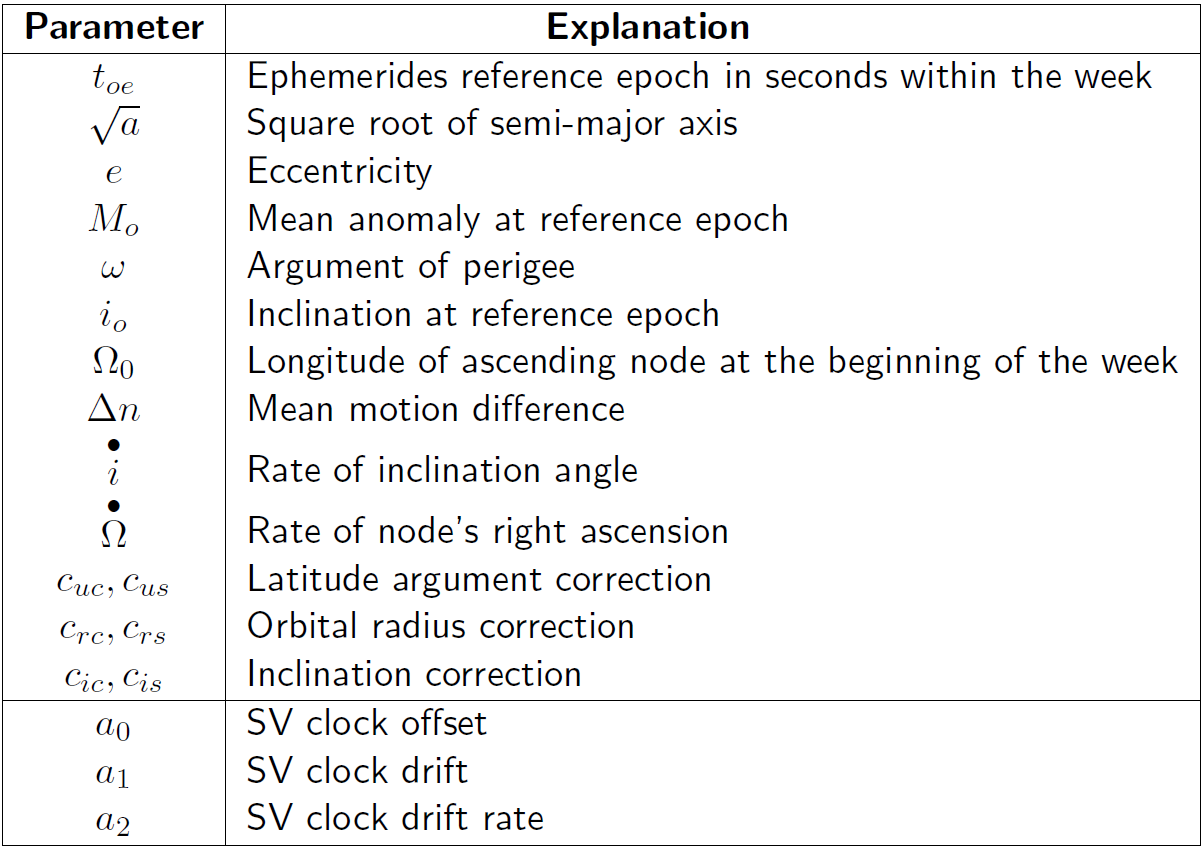If you wish to contribute or participate in the discussions about articles you are invited to contact the Editor

# Difference between revisions of "GPS and Galileo Satellite Coordinates Computation"Fundamentals
Title GPS and Galileo Satellite Coordinates Computation
Author(s) J. Sanz Subirana, J.M. Juan Zornoza and M. Hernández-Pajares, Technical University of Catalonia, Spain.
Level Intermediate
Year of Publication 2011

Table 1 provides the GPS or Galileo broadcast ephemeris parameters to compute their satellite coordinates at any observation epoch. These parameters are periodically renewed (typically every 2 hours for GPS and 3 hours for Galileo) and must not be used out of the prescribed time (about four hours), because the extrapolation error grows exponentially beyond its validity period.

The algorithm provided is from the [IS-GPS-200, table 20-IV] [footnotes 1].The Galileo satellites follow a similar schemeTable 1: GPS and Galileo broadcast ephemeris and clock message parameters.

In order to compute satellite coordinates from navigation message, the algorithm provided as follows must be used. An accuracy of about 4 meters (RMS) is achieved for GPS satellites with S/A=0ff and several tens of meters with S/A=on [footnotes 2]:

• Compute the time $t_k$ from the ephemerides reference epoch $t_{oe}$ ($t$ and $t_{oe}$ are expressed in seconds in the GPS week):
$t_k=t-t_{oe}$
If $t_k\gt 302\,400$ sec, subtract $604\,800$ sec from $t_k$. If $t_k\lt -302\,400$ sec, add $604\,800$ sec.

• Compute the mean anomaly for $t_k$,
$M_k=M_o+\left( \frac{\sqrt{\mu }}{\sqrt{a^3}}+\Delta n\right)t_k$

• Solve (iteratively) the Kepler equation for the eccentricity anomaly $E_k$:
$M_k=E_k-e\sin E_k$

• Compute the true anomaly $v_k$:
$v_k=\arctan \left( \frac{\sqrt{1-e^2}\sin E_k}{\cos E_k-e}\right)$

• Compute the argument of latitude $u_k$ from the argument of perigee $\omega$, true anomaly $v_k$ and corrections $c_{uc}$ and $c_{us}$:
$u_k=\omega +v_k+c_{uc}\cos 2\left( \omega +v_k\right) +c_{us}\sin 2\left( \omega +v_k\right)$

• Compute the radial distance $r_k$, considering corrections $c_{rc}$ and $c_{rs}$:
$r_k=a\left( 1-e\cos E_k\right) +c_{rc}\cos 2\left( \omega +v_k\right) +c_{rs}\sin 2\left( \omega +v_k\right)$

• Compute the inclination $i_k$ of the orbital plane from the inclination $i_o$ at reference time $t_{oe}$, and corrections $c_{ic}$ and $c_{is}$:
$i_k=i_o+\stackrel{\bullet }{i} t_k+c_{ic}\cos 2\left( \omega +v_k\right) +c_{is}\sin 2\left( \omega +v_k\right)$

• Compute the longitude of the ascending node $\lambda_k$ (with respect to Greenwich). This calculation uses the right ascension at the beginning of the current week ($\Omega _o$), the correction from the apparent sidereal time variation in Greenwich between the beginning of the week and reference time $t_k=t-toe$, and the change in longitude of the ascending node from the reference time $t_{oe}$:
$\lambda _k=\Omega _o+\left( \stackrel{\bullet }{\Omega }-\omega _E\right) t_k-\omega _E t_{oe}$

• Compute the coordinates in TRS frame, applying three rotations (around $u_k$, $i_k$ and $\lambda _k$):
$\left[ \begin{array}{c} X_k \\ Y_k \\ Z_k \end{array} \right] ={\mathbf R}_3\left( -\lambda _k\right) {\mathbf R}_1\left( -i_k\right) {\mathbf R}_3\left( -u_k\right) \left [ \begin{array}{c} r_k \\ 0 \\ 0 \end{array} \right]$

where ${\mathbf R}_1$ and ${\mathbf R_3}$ are the rotation matrices defined in Transformation between Terrestrial Frames.